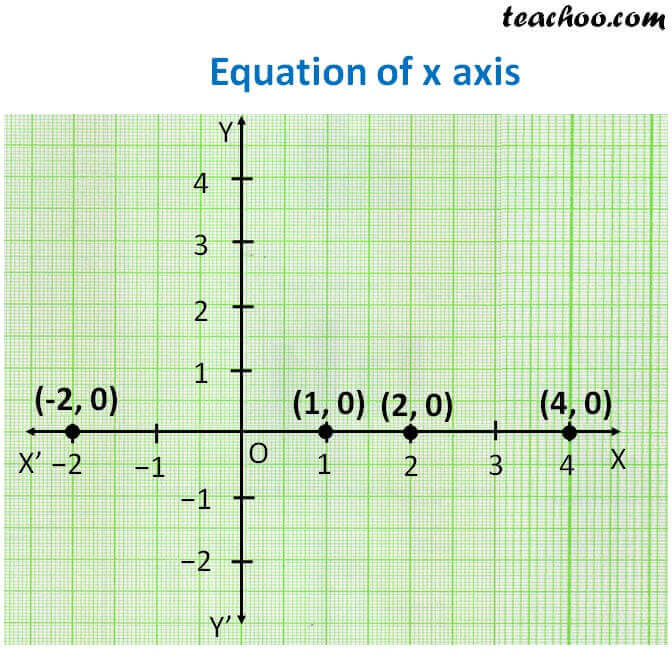Lines parallel x or y axis

Chapter 4 Class 9 Linear Equations in Two Variables
Concept wise

Let us plot points (1, 0) (2, 0) (4, 0) (−2, 0) on the graphAll points in x-axis are like

(1, 0) (2, 0) (4, 0) (−2, 0)

So y = 0 for all points in x − axis

∴ Equation of x axis is y = 0

Learn in your speed, with individual attention - Teachoo Maths 1-on-1 Class